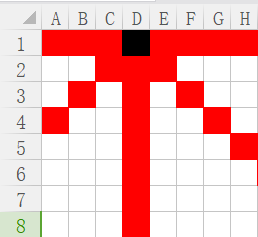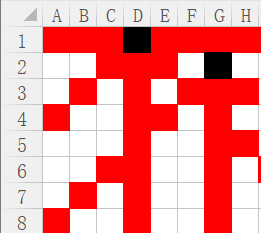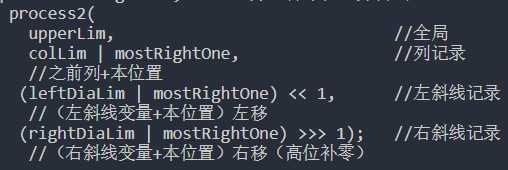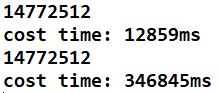# 皇后问题

（本文不展开讲状态压缩，以后再说）

# 一般思路：

N*N的二维数组，在每一个位置进行尝试，在当前位置上判断是否满足放置皇后的条件（这一点的行、列、对角线上，没有皇后）。

# 优化1：

public class NQueens {
public static int num1(int n) {
if (n < 1) {
return 0;
}
int[] record = new int[n];
return process1(0, record, n);
}
public static int process1(int i, int[] record, int n) {
if (i == n) {
return 1;
}
int res = 0;
for (int j = 0; j < n; j++) {
if (isValid(record, i, j)) {
record[i] = j;
res += process1(i + 1, record, n);
}
}//对于当前行，依次尝试每列
return res;
}
//判断当前位置是否可以放置
public static boolean isValid(int[] record, int i, int j) {
for (int k = 0; k < i; k++) {
if (j == record[k] || Math.abs(record[k] - j) == Math.abs(i - k)) {
return false;
}
}
return true;
}
public static void main(String[] args) {
int n = 8;
System.out.println(num1(n));
}
}


# 优化2：public class NQueens {
public static int num2(int n) {
// 因为本方法中位运算的载体是int型变量，所以该方法只能算1~32皇后问题
// 如果想计算更多的皇后问题，需使用包含更多位的变量
if (n < 1 || n > 32) {
return 0;
}
int upperLim = n == 32 ? -1 : (1 << n) - 1;
//upperLim的作用为棋盘大小，比如8皇后为00000000 00000000 00000000 11111111
//32皇后为11111111 11111111 11111111 11111111
return process2(upperLim, 0, 0, 0);
}

public static int process2(int upperLim, int colLim, int leftDiaLim,
int rightDiaLim) {
if (colLim == upperLim) {
return 1;
}
int pos = 0;            //pos：所有的合法位置
int mostRightOne = 0;   //所有合法位置的最右位置

//所有记录按位或之后取反，并与全1按位与，得出所有合法位置
pos = upperLim & (~(colLim | leftDiaLim | rightDiaLim));
int res = 0;//计数
while (pos != 0) {
mostRightOne = pos & (~pos + 1);//取最右的合法位置
pos = pos - mostRightOne;       //去掉本位置并尝试
res += process2(
upperLim,                             //全局
colLim | mostRightOne,                //列记录
//之前列+本位置
(leftDiaLim | mostRightOne) << 1,      //左斜线记录
//（左斜线变量+本位置）左移
(rightDiaLim | mostRightOne) >>> 1);   //右斜线记录
//（右斜线变量+本位置）右移（高位补零）
}
return res;
}

public static void main(String[] args) {
int n = 8;
System.out.println(num2(n));
}
}


32皇后：结果/时间public class NQueens {

public static int num1(int n) {
if (n < 1) {
return 0;
}
int[] record = new int[n];
return process1(0, record, n);
}

public static int process1(int i, int[] record, int n) {
if (i == n) {
return 1;
}
int res = 0;
for (int j = 0; j < n; j++) {
if (isValid(record, i, j)) {
record[i] = j;
res += process1(i + 1, record, n);
}
}
return res;
}

public static boolean isValid(int[] record, int i, int j) {
for (int k = 0; k < i; k++) {
if (j == record[k] || Math.abs(record[k] - j) == Math.abs(i - k)) {
return false;
}
}
return true;
}

public static int num2(int n) {
if (n < 1 || n > 32) {
return 0;
}
int upperLim = n == 32 ? -1 : (1 << n) - 1;
return process2(upperLim, 0, 0, 0);
}

public static int process2(int upperLim, int colLim, int leftDiaLim,
int rightDiaLim) {
if (colLim == upperLim) {
return 1;
}
int pos = 0;
int mostRightOne = 0;
pos = upperLim & (~(colLim | leftDiaLim | rightDiaLim));
int res = 0;
while (pos != 0) {
mostRightOne = pos & (~pos + 1);
pos = pos - mostRightOne;
res += process2(upperLim, colLim | mostRightOne,
(leftDiaLim | mostRightOne) << 1,
(rightDiaLim | mostRightOne) >>> 1);
}
return res;
}

public static void main(String[] args) {
int n = 32;

long start = System.currentTimeMillis();
System.out.println(num2(n));
long end = System.currentTimeMillis();
System.out.println("cost time: " + (end - start) + "ms");

start = System.currentTimeMillis();
System.out.println(num1(n));
end = System.currentTimeMillis();
System.out.println("cost time: " + (end - start) + "ms");
}
}


11-086万+

#### 八皇后问题详解(四种解法)09-212万+

#### n皇后问题

10-121万+

#### 算法总结——八皇后问题（三种解法）

01-287314

#### 八皇后问题（递归回溯算法详解+C代码）

08-051701

#### 8皇后问题详细过程

03-246951

#### 八皇后问题详解

09-234万+

#### 8皇后问题（java算法实现）

07-033万+

#### n皇后问题-回溯法求解

01-152万+

#### N皇后问题©️2020 CSDN 皮肤主题: 书香水墨 设计师: CSDN官方博客点击重新获取扫码支付1.余额是钱包充值的虚拟货币，按照1:1的比例进行支付金额的抵扣。
2.余额无法直接购买下载，可以购买VIP、C币套餐、付费专栏及课程。余额充值